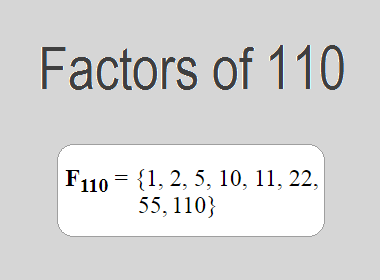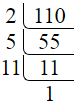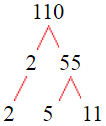# Factors of 110The factors of 110 are 1, 2, 5, 10, 11, 22, 55, and 110 i.e. F110 = {1, 2, 5, 10, 11, 22, 55, 110}. The factors of 110 are all the numbers that can divide 110 without leaving a remainder.

We can check if these numbers are factors of 110 by dividing 110 by each of them. If the result is a whole number, then the number is a factor of 110. Let's do this for each of the numbers listed above:

·        1 is a factor of 110 because 110 divided by 1 is 110.

·        2 is a factor of 110 because 110 divided by 2 is 55.

·        5 is a factor of 110 because 110 divided by 5 is 22.

·        10 is a factor of 110 because 110 divided by 10 is 11.

·        11 is a factor of 110 because 110 divided by 11 is 10.

·        22 is a factor of 110 because 110 divided by 22 is 5.

·        55 is a factor of 110 because 110 divided by 55 is 2.

·        110 is a factor of 110 because 110 divided by 110 is 1.

## How to Find Factors of 110?

1 and the number itself are the factors of every number. So, 1 and 110 are two factors of 110. To find the other factors of 110, we can start by dividing 110 by the numbers between 1 and 110. If we divide 110 by 2, we get a remainder of 0. Therefore, 2 is a factor of 110. If we divide 110 by 3, we get a remainder of 2. Therefore, 3 is not a factor of 110.

Next, we can check if 4 is a factor of 110. If we divide 110 by 4, we get a remainder of 2. Therefore, 4 is not a factor of 110. We can continue this process for all the possible factors of 110.

Through this process, we can find that the factors of 110 are 1, 2, 5, 10, 11, 22, 55, and 110. These are the only numbers that can divide 110 without leaving a remainder.

********************

********************

## Properties of the Factors of 110

The factors of 110 have some interesting properties. One of the properties is that the sum of the factors of 110 is equal to 216. We can see this by adding all the factors of 110 together:

1 + 2 + 5 + 10 + 11 + 22 + 55 + 110 = 216

Another property of the factors of 110 is that the prime factors of 110 are 2, 5, and 11 only.

## Applications of the Factors of 110

The factors of 110 have several applications in mathematics. One of the applications is in finding the highest common factor (HCF) of two or more numbers. The HCF is the largest factor that two or more numbers have in common. For example, to find the HCF of 110 and 33, we need to find the factors of both numbers and identify the largest factor they have in common. The factors of 110 are 1, 2, 5, 10, 11, 22, 55, and 110. The factors of 33 are 1, 3, 11, and 33. The largest factor that they have in common is 11. Therefore, the HCF of 110 and 33 is 11.

Another application of the factors of 110 is in prime factorization. Prime factorization is the process of expressing a number as the product of its prime factors. The prime factors of 110 are 2, 5, and 11 since these are the only prime numbers that can divide 110 without leaving a remainder. Therefore, we can express 110 as:

110 = 2 × 5 × 11

We can do prime factorization by division and factor tree method also. Here is the prime factorization of 110 by division method,110 = 2 × 5 × 11

Here is the prime factorization of 110 by the factor tree method,110 = 2 × 5 × 11

## Conclusion

The factors of 110 are the numbers that can divide 110 without leaving a remainder. The factors of 110 are 1, 2, 5, 10, 11, 22, 55, and 110. The factors of 110 have some interesting properties, such as having a sum of 216. The factors of 110 have several applications in mathematics, such as finding the highest common factor and prime factorization.